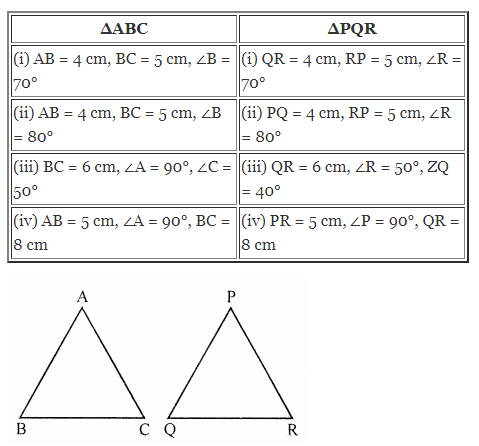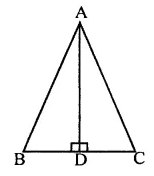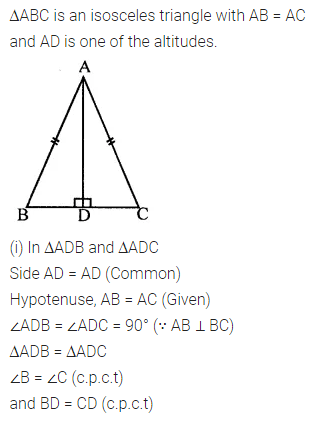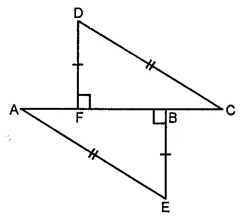# ML Aggarwal Class 7 Solutions for ICSE Maths Chapter 12 Congruence of Triangles Check Your Progress

## ML Aggarwal Class 7 Solutions for ICSE Maths Chapter 12 Congruence of Triangles Check Your Progress

Question 1.
State, giving reasons, whether the following pairs of triangles are congruent or not:Solution:Question 2.
Given below are measurements of some parts of two triangles. Examine whether the two triangles are congruent or not. In case of congruence, give reasons and write in symbolic form:Solution:Question 3.
In the given figure, ABC is an isosceles triangle with AB = AC and AD is one of its altitudes.
(i) State the three pairs of equal parts in ∆ADB and ∆ADC.
(iii) Is ∠B = ∠C? Why?
(iv) Is BD = DC? Why?Solution:Question 4.
In the given figure, OA bisects ∠A and ∠ABO = ∠OCA. Prove that OB = OC.Solution:Question 5.
In the given figure , prove that
(i) AB = FC
(ii) AF = BC.Solution: